# C Program to Add Two Matrices

In this article, you will learn about adding two matrices in C. But before going through the program, if you are not aware of how two matrices get added, then I recommend that you refer to the Matrix Addition article. There, it will take very little time to see the pictorial representation of how matrix addition goes.

To add any two matrices in C programming, you have to ask the user to enter all elements of both matrices. Now start adding the two matrices together to form a new matrix. After adding two matrices, display the third matrix, which is the addition result of the two matrices given by the user, as shown in the program given here.

```#include<stdio.h>
#include<conio.h>
int main()
{
int mat1, mat2, i, j, mat3;
printf("Enter 3*3 matrix 1 elements :");
for(i=0; i<3; i++)
{
for(j=0; j<3; j++)
scanf("%d",&mat1[i][j]);
}
printf("Enter 3*3 matrix 2 elements :");
for(i=0; i<3; i++)
{
for(j=0; j<3; j++)
scanf("%d",&mat2[i][j]);
}
for(i=0; i<3; i++)
{
for(j=0; j<3; j++)
mat3[i][j]=mat1[i][j]+mat2[i][j];
}
printf("\nHere is the new matrix:\n");
for(i=0; i<3; i++)
{
for(j=0; j<3; j++)
printf("%d ",mat3[i][j]);
printf("\n");
}
getch();
return 0;
}```

Because the above program was written in the Code::Blocks IDE, here is a sample run for better comprehension. This is the first snapshot of the sample run: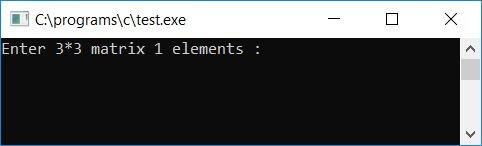Now, fill the first 3*3 matrix with any 9 elements and the second 3*3 matrix with any 9 elements.Press the ENTER key to see the output. The output will be the addition result of the given two matrices, as shown here in the second snapshot of the sample run: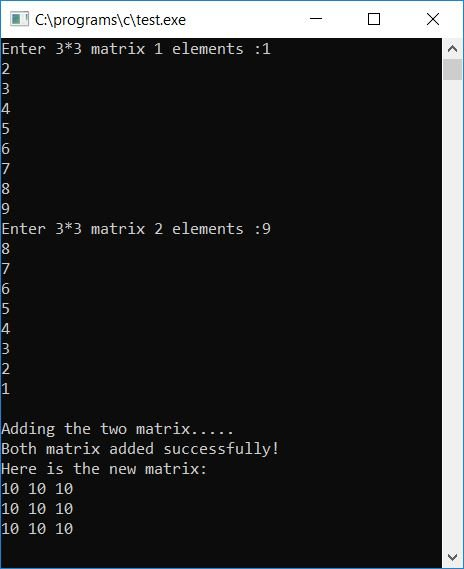#### Program Explained

1. Taking nine elements from the first matrix using a for loop
2. First, i begins with 0 and j with 0.
3. Therefore, the first element is initialized to mat1.
4. Now that j gets incremented and becomes 1, i still holds the value 0.
5. Therefore, the second element is initialized to mat1.
6. In the same way, the third element is initialized to mat1.
7. When j increases to 3, then the condition j<3 evaluates to false.
8. Therefore, the compiler skip this loop and goes to the outer loop.
9. And it increases the value of i.
10. Because i is currently 0, it will becomes 1 after the increment.
11. The compiler enters the inner loop once more and sets j to 0.
12. Now the same processes starts again that was from step no. 2 to step no. 7, but here i holds 1, not 0.
13. That is, the fourth, fifth, and sixth elements are initialized to mat1, mat1, and mat1.
14. Similarly, the rest of the elements in matrix 1 and matrix 2 are initialized.
15. To add the matrix, we have to use a similar corresponding index number for both the matrices, like mat1 + mat2 will be added and initialized to the third matrix mat3. mat1 + mat2 will be added and initialized to the third matrix mat3, etc.
16. In a similar way, do this for all the 9 elements and initialize to corresponding index of the third matrix.
17. Finally, print the value of the third matrix as output.

### Add two matrices of the given size in C

Let's modify the above program with some extra features. The additional feature is that the user can specify the size of the matrix.

```#include<stdio.h>
#include<conio.h>
int main()
{
int x, y, i, j;
printf("Enter the size of matrix:-\n");
printf("Enter row size:");
scanf("%d",&x);
printf("Enter column size:");
scanf("%d",&y);
printf("\nEnter elements of first matrix:");
for(i=0; i<x; i++)
{
for(j=0; j<y; j++)
scanf("%d", &mat1[i][j]);
}
printf("Enter elements of second matrix:");
for(i=0; i<x; i++)
{
for(j=0; j<y; j++)
scanf("%d", &mat2[i][j]);
}
for(i=0; i<x; i++)
{
for(j=0; j<y; j++)
}
for(i=0; i<x; i++)
{
for(j=0; j<y; j++)
printf("\n");
}
getch();
return 0;
}```

This is the first screenshot of the sample run: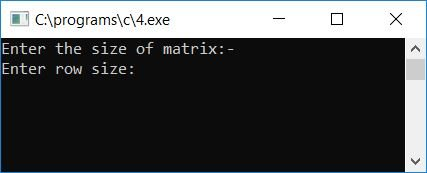This is the second screenshot after providing row and column sizes: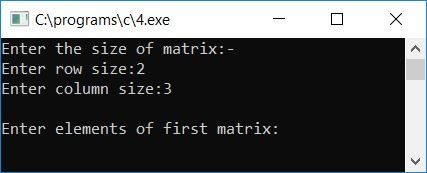And this is the third screenshot after providing 2*3 matrix elements for both matrices: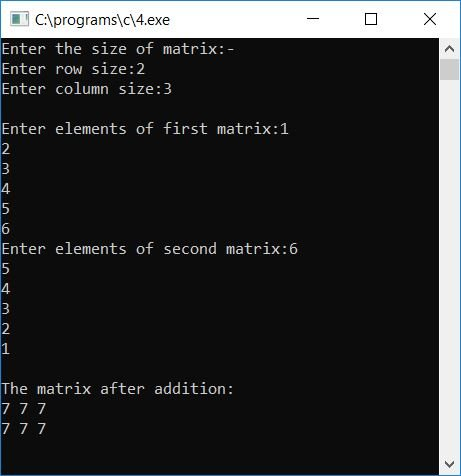#### The same program in different languages

C Quiz

« Previous Program Next Program »